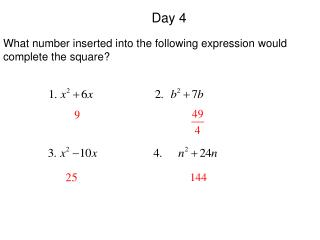DownloadDownload PresentationDay 4

# Day 4

Télécharger la présentation## Day 4

- - - - - - - - - - - - - - - - - - - - - - - - - - - E N D - - - - - - - - - - - - - - - - - - - - - - - - - - -
##### Presentation Transcript

1. Day 4 What number inserted into the following expression would complete the square?

2. 10.6 Using the Quadratic Formula I can use the quadratic formula to solve a quadratic equation.

3. 10.6 Using the Quadratic Formula Methods for Solving Quadratic Equations Factor Square Root Completing the Square Quadratic Formula Quadratic Formula: Used to solve any kind of quadratic equation.

4. Rule for Using the Quadratic Formula The equation must be in standard form, It will be very helpful to list out a = , b = , and c = each time you use the formula to minimize your mistakes.

5. Solve. Ex. 1

6. Solve. Ex. 2

7. Solve. Ex. 3

8. Solve. Ex. 4

9. Suppose a football player kicks a ball and gives it an initial upward velocity of 47ft/s. The starting height of the football is 3ft. If no one catches the football, how long will it be in the air? Ex. 5 NOTE: For your homework tomorrow night, DO NOT answer the question. I want you to draw a sketch of what you think this picture would look like on a graph. Tell me what the x-axis symbolizes, what the y-axis symbolizes, and what the zeros represent in the problem. What does the x-axis stand for? What does the y-axis stand for? What do the zeros represent? Time the ball is in the air Height of the ball The amount of time it takes the ball to hit the ground.